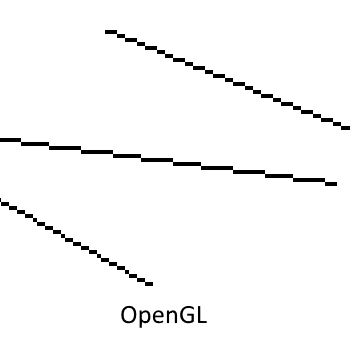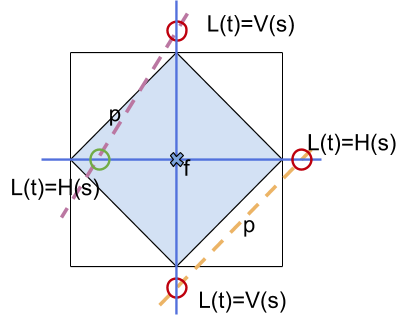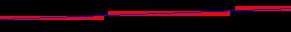# OpenGL Line Segment Rasterization

OpenGL and Vulkan both render line segments as a series of pixels between two points. They differ in which pixels cover the line.

For single sample rendering Vulkan uses an algorithm based on quad coverage. A small shape is extruded around the line segment. Samples covered by the shape then represent the line segment. See the Vulkan spec for more details.

OpenGL‘s algorithm is based on Bresenham's line algorithm. Bresenham’s algorithm selects pixels on the line between the two segment points. Note Bresenham's does not support multisampling. When compared visually you can see the Vulkan line segment rasterization algorithm always selects a superset of the line segment pixels rasterized in OpenGL. See this example:The OpenGL spec defines a “diamond-exit” rule to select fragments on a line. Please refer to the 2.0 spec section 3.4.1 “Basic Line Segment Rasterization” spec for more details. To implement this rule we inject a small computation to test if a pixel falls within the diamond in the start of the pixel shader. If the pixel fails the diamond test we discard the fragment. Note that we only perform this test when drawing lines. See the section on Shader Compilation for more info. See the below diagram for an illustration of the diamond rule:The diamond rule can be implemented in the fragment shader by computing the intersection between the line segment and the grid that crosses the pixel center. If the distance between an intersection and the pixel center is less than half a pixel then the line enters and exits the diamond. `f` is the pixel center in the diagram. The green circle indicates a diamond exit and the red circles indicate intersections that do not exit the diamond. We detect non-Bresenham fragments when both intersections are outside the diamond.

The full code derivation is omitted for brevity. It produces the following fragment shader patch implementation:

```vec2 p = (((((ANGLEPosition.xy) * 0.5) + 0.5) * viewport.zw) + viewport.xy);
vec2 d = dFdx(p) + dFdy(p);
vec2 f = gl_FragCoord.xy;
vec2 p_ = p.yx;
vec2 d_ = d.yx;
vec2 f_ = f.yx;

vec2 i = abs(p - f + (d/d_) * (f_ - p_));

if (i.x > 0.500001 && i.y  > 0.500001)
discard;
```

Note that we must also pass the viewport size as an internal uniform. We use a small epsilon value to correct for cases when the line segment is perfectly parallel or perpendicular to the window. For code please see TranslatorVulkan.cpp under `AddLineSegmentRasterizationEmulation`.

## Limitations

Although this emulation passes all current GLES CTS tests it is not guaranteed to produce conformant lines. In particular lines that very nearly intersect the junction of four pixels render with holes. For example:Therefore for a complete implementation we require the Bresenham line rasterization feature from VK_EXT_line_rasterization.